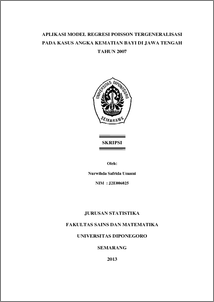# APLIKASI MODEL REGRESI POISSON TERGENERALISASI PADA KASUS ANGKA KEMATIAN BAYI DI JAWA TENGAH TAHUN 2007

Umami, Nurwihda Safrida (2013) APLIKASI MODEL REGRESI POISSON TERGENERALISASI PADA KASUS ANGKA KEMATIAN BAYI DI JAWA TENGAH TAHUN 2007. Undergraduate thesis, UNIVERSITAS DIPONEGORO.Preview
PDF
497Kb

## Abstract

Infant Mortality is one of the issues that can affect the number and age composition of the population. The Government pays special attention to reduce the amount of Infant Mortality Rate in Central Java, so the role of data and information becomes very important. Poisson regression is a nonlinear regression which is often used to model the relationship between the response variable in the form of discrete data with predictor variables in the form of continuous or discrete data. Poisson regression models have equidispersi assumption, a condition in which the mean and variance of the response variable have equal value. In practice, the assumption is sometimes violated in the analysis of discrete data in the form of underdispersi or overdispersi so that Poisson regression model is not appropriate to be used. Overdispersi is a condition in which the data of response variable shows the value of the variance that is greater than the mean value. One model that can be used to solve the overdispersi problem is to use generalized Poisson regression model. The regression model is an extension of the Poisson regression and part of the Generalized Linear Model (GLM) which does not require constancy of variance to test the hypothesis. From the data of Infant Mortality Rate in Central Java in 2007 known that there overdispersi be addressed using generalized Poisson regression models. And the result, factors affecting Infant Mortality Rate in Central Java in 2007 is the number of health facilities, the number of medical personnel, and the percentage of households with clean water each county / city. Keywords: Infant Mortality Rate, Poisson Regression, Overdispersion, Generalized Poisson Regression, AIC

Item Type: Thesis (Undergraduate) H Social Sciences > HA Statistics Faculty of Science and Mathematics > Department of Statistics 42582 Mr Hasbi Yasin 03 Mar 2014 16:22 03 Mar 2014 16:22

Repository Staff Only: item control page